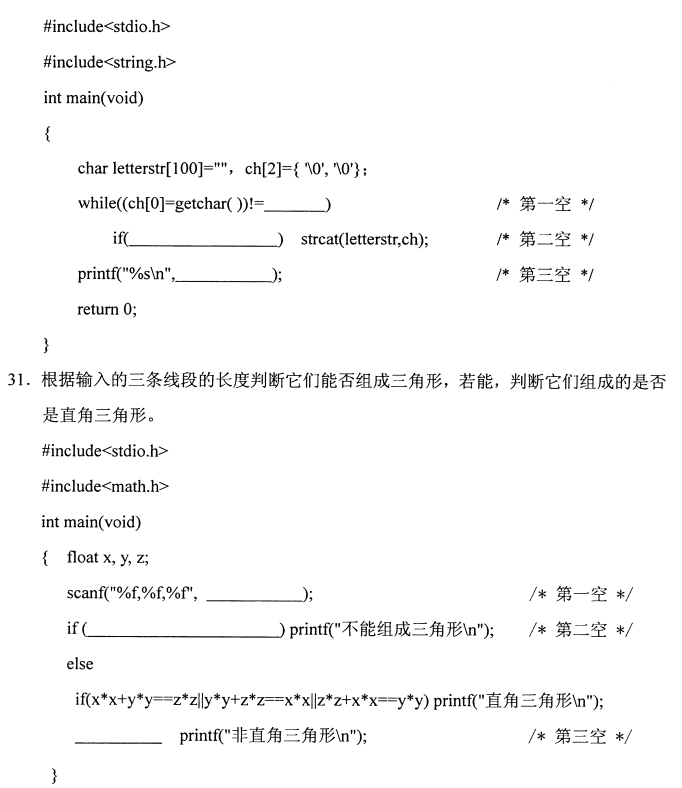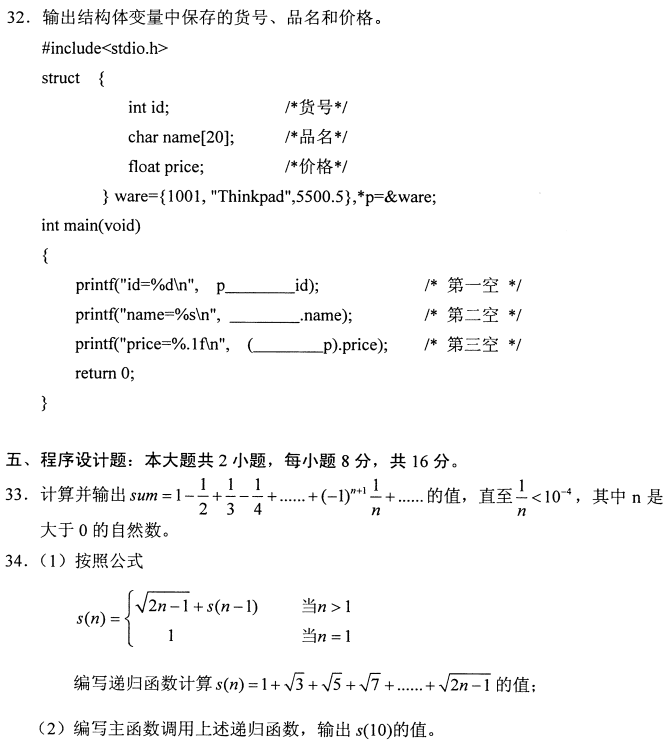# 内蒙古自考2021年10月高级语言程序设计(一)真题

1.请考生按规定用笔将所有试题的答案涂、写在答题纸上。

2.答题前,考生务必将自己的考试课程名称、姓名、准考证号用黑色字迹的签字笔或钢笔填写在答题纸规定的位置上。

1.下列不是程序设计语言的是

A. C语言

B. BASIC 语言

C. JAVA语言

D.自然语言

2.-下列为 C语言关键字的是

A. break

B. BREAK

C. File

D. printf

3.正确的整型常量是

A.2e3

B.0XAB

C. '12'

D.089

4.设int a=0, b=0, m=1;,则表达式(a!=b)&&(m=2)和m的值分别是

A.0，1

B.1，1

C.0，2

D.1，2

5.设int a={3,2,1};，下列语句输出结果为0的是

A. printf("%d", a);

B. printf("%d", a);

C. print("%d", a);

D. print("%d", a);

6.设inta,b;, 若从键盘分别输入a和b的值，则正确的输入语句是

A. scanf("%d,%d",a,b);

B. scanf("%f,%f",a,b);

C. scanf("%d,%d" ,&a,&b);

D. scanf("%f,%f",&a,&b);

7.执行语句print("%d\n",1&2);后输出结果是

A. -1

B.0

C.1

D. 2

8.设intp;， 与if (p==0) 等价的是

A. if(p)

B. if(!p)

C. if(p=1)

D. if(p!=0)

9.设int a=1;，下 列语句中循环体执行次数为1的是

A. for( ; a<1; a++) printf("%d\n",a);

B. for(; a>0; a++) pritf("%d\n",a);

C. while(a<=1) printf("%d\n" ,a++);

D. while(a> 1) printf("%d\n" ,a++);

10.设int a[ ]={{1},{2,3}};,则数组元素a的值是

A.0

B.1

C.2

D.3

11.设char s[ ]="China";，则sizeof(s)和strlen (s)的值分别是

A.5和5

B.5和6

C.6和5

D.6和6

12.设struct { int x; char y;} z={{1, 'A'},{2, 'B',{3, 'C'}};,表达式zx+z.y的值是

A.66

B.67

C.68

D.69

13.设int f(float a, double b,char c);，则函数f返回值的类型是

A. int 型

B. char 型

C. float 型

D. double 型

14.设inti=l,j=0,*p=&i,*q=&j;，则与j=i;等价的是

A. j=p;

B. j=q;

C. j=*p;

D. j=*q;

15.设char *ps[ ]={"How are you?", "Fine.l0 Thank you."};,执行语句printf("%s %s\n",ps,ps);后输出结果是

A. How are you?

B. How are you? Fine.

C. Fine.Thank you.

D. How are you? Fine.Thank you.

16. C语言源程序经过编译后，生成的是           程序。

17.结构化程序的三种基本结构分别是顺序结构、选择结构和           结构。

18.C语言中，十六进制整型常量必须以           开头。

19. 设inta, *p=&a;， 则*p与p中的内容分别是a的

20.设inta=1;，执行printf("%d\n" ,a++);的输出结果是

21.设int s[ ]={1,2,3,4,5};，则数组s的行长度至少是

22.若调用数学函数sqrt()，则需要使用#include命令包含头文件

23.设int main(void) { int a; ....变量a的默认存储类型是

24.设FILE *fp;，以只读方式打开二进制文件的语句是fp=fopen("a.dat",           );。

25. C语言中，宏定义命令是

int main(void)

{

int sum=15, i-1;

for(; i<10;i+)

{

if（sum>20） break；

if（i%2——0）

{    sum+-i; printf("%d, ",sum);    }

}

print("i-%dn",i);

return 0:

}

int main(void)

{

int a-{1,3, 2, 4}, b-{0,2, 1,3};

int c-{0, 0, 0,0}, i,j;

for (i=0; i<=1; i++)

for （j=0；j<=1；j++） c[i][j]=a[i]*b[j]+a[i]*b[j]；

printf（“%d\n”， c*c-c*c）；

return 0;

}

long FUN(int n)

{

if(n==1|n= =2) return (1L);

else return (FUN(n-1)+FUN(n-2));

}

int main(void)

{

int i;

for (i-1;i<4;i+) print("%ld,",FUN(i));

print("%1d\n",FUN(4));

return 0:

}

int fun(int x,int y,int z)

{

int max;

max=x<y?y:x;

if(z>max) max=z;

return max;

}

int main(void)

{

int a=-7,b=3,c=3,d=5,e=-4;

printf("%d\n",fun(fun(a,b,c),d,e));

return 0;

}

30.从键盘输入一串字符，以回车换行为结束标志，将其中的小写英文字母联成一个新的字符串并输出。1、由于各方面情况的调整与变化，本网提供的考试信息仅供参考，考试信息以省考试院及院校官方发布的信息为准。

2、本网信息来源为其他媒体的稿件转载，免费转载出于非商业性学习目的，版权归原作者所有，如有内容与版权问题等请与本站联系。

### 微信交流群﻿